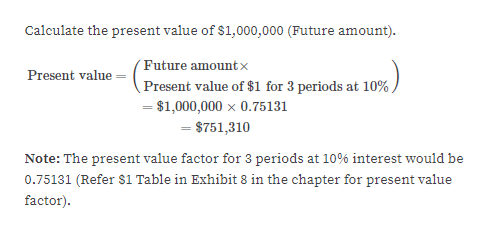# Tommy John is going to receive \$1,000,000 in three years. The current market rate of interest is 10%.a. Using the present value of \$1 table in Exhibit 8, determine the present value of this amount compounded annually.b. Why is the present value less than the \$1,000,000 to be received in the future?

Question
1 views

Tommy John is going to receive \$1,000,000 in three years. The current market rate of interest is 10%.
a. Using the present value of \$1 table in Exhibit 8, determine the present value of this amount compounded annually.
b. Why is the present value less than the \$1,000,000 to be received in the future?

check_circle

Step 1

a.

determine the present value of this ...help_outlineImage TranscriptioncloseCalculate the present value of \$1,000,000 (Future amount). (Future amount x Present value of \$1 for 3 periods at 10% = \$1,000,000 × 0.75131 = \$751,310 Present value Note: The present value factor for 3 periods at 10% interest would be 0.75131 (Refer \$1 Table in Exhibit 8 in the chapter for present value factor). fullscreen

### Want to see the full answer?

See Solution

#### Want to see this answer and more?

Solutions are written by subject experts who are available 24/7. Questions are typically answered within 1 hour.*

See Solution
*Response times may vary by subject and question.
Tagged in